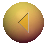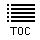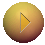Gravity: Space as a Rubber Sheet
Slide 5 of 22Go to slide:

Think of space as a stretched rubber sheet. When something heavy is placed on the sheet, it causes it to dip. The heavier the object, the deeper the resulting gravitational well. In the words of John Wheeler "matter tells space how to curve". Once one accepts the curvature of space, it is rather easy to see that smaller objects will move along the straightest possible line that they can in that curved space. However, this straightest possible line has different properties than in flat space. In fact, the line itself looks curved, as shown above. Again in the words of Wheeler, the curved space tells the matter how to move. In curved space the rules of Euclidean geometry are no longer valid. For example, it is possible for parallel, straight lines to meet: think of the lines of longitude on a the curved surface of a spherical globe: they are parallel at the equator, straight and meet at the poles. Also, the sum of angles in a triangle does not have to equal 180 degrees: think of the triangle made by two lines of longitude that meet at the north pole, and the segment of the equator joining them. This triangle has two right angles at its base (the equator), and a non-zero angle at the apex (the north pole). Hence the sum is greater than 180. Once space itself is curved, everything moving in it is affected. Thus not only particles, but light too must feel the effects of gravity.